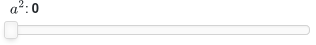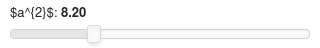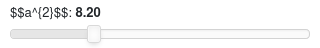# Latex on slider's label when using params

I’m trying panel v1.1.0. Latex labels works fine when instantiating a panel’s widget, for example:

``````import panel as pn
import param
pn.extension()

pn.widgets.FloatSlider(name="\$\$a^{2}\$\$").servable().show()
``````However, they don’t render when I’m using a `Parameterized` class:

``````class BaseClass(param.Parameterized):
float_with_hard_bounds  = param.Number(default=8.2, bounds=(7.5, 10), label="\$a^{2}\$")

pn.Param(BaseClass.param.float_with_hard_bounds).servable().show()
``````Is there some way to get it to work in the second case?

No, I tried it before:This is a Bokeh bug, when wrapping sliders into column/rows.

Example in Panel:

``````import panel as pn
pn.Column(pn.widgets.FloatSlider(name="\$\$a^{2}\$\$")).servable()
``````

Example in Bokeh:

``````from bokeh.io import curdoc
from bokeh.layouts import column
from bokeh.models.widgets import Slider

slider = Slider(start=0, end=10, value=1, step=0.1, title="\$\$a^{2}\$\$")
c = column(children=[slider])
A small note you shouldn’t use `.servable().show()` you should use one of them:
• `.servable()` with `panel serve filename`
• `.show()` with `python filename`